##### Trigonometry Workbook For DummiesYou can transform any function into a related function by shifting it horizontally or vertically, flipping it over (reflecting it) horizontally or vertically, or stretching or shrinking it horizontally or vertically. Let’s go through the horizontal transformations. Consider the exponential function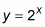Take a look at the following graph.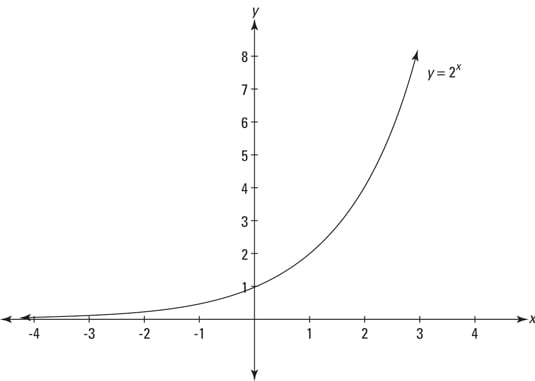You make horizontal changes by adding a number to or subtracting a number from the input variable x, or by multiplying x by some number.

All horizontal transformations, except reflection, work the opposite way you’d expect:

• Adding to x makes the function go left.

• Subtracting from x makes the function go right.

• Multiplying x by a number greater than 1 shrinks the function.

• Multiplying x by a number less than 1 expands the function.

## Horizontal translation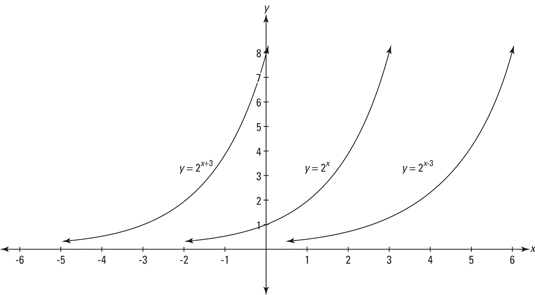For example, the graph of y = 2x+3 has the same shape and orientation as the graph for y = 2x. It’s just shifted three units to the left. Instead of passing through (0, 1) and (1, 2), the shifted function goes through (–3, 1) and (–2, 2). And the graph of y = 2x–3 is three units to the right of y = 2x.

## Horizontal shrinking and stretching

For the next two transformations, why don’t you try graphing them on your own.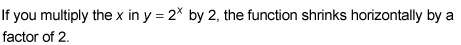So, every point on the new function is half of its original distance from the y-axis. The y-coordinate of every point stays the same; the x-coordinate is cut in half. For example,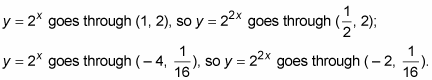Multiplying x by a number less than 1 has the opposite effect.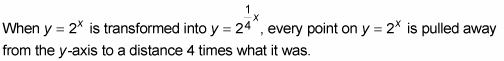## Horizontal reflection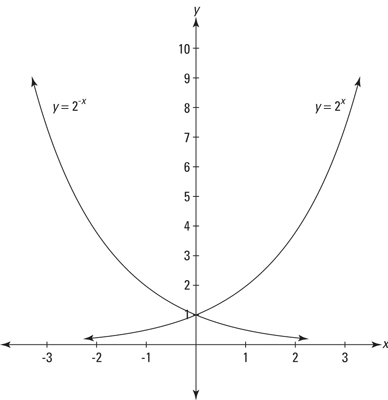The last horizontal transformation is a reflection over the y-axis.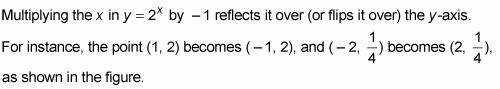Note that after the reflection, points are on the opposite side of the y-axis, but remain the same distance from the axis. And original points that lie on the y-axis (the y-intercepts) stay where they are.